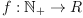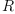login  home  contents  what's new  discussion  bug reports help  links  subscribe  changes  refresh  edit

Drichlet ring is now included in FriCAS

The Dirichlet Ring

The Dirichlet Ring is the ring of arithmetical functions(see http://en.wikipedia.org/wiki/Arithmetic_function) together with the Dirichlet convolution (see http://en.wikipedia.org/wiki/Dirichlet_convolution) as multiplication and component-wise addition. Since we can consider the values an arithmetic functions assumes as the coefficients of a Dirichlet generating series, we callthe coefficient ring of a function.

In general we only assume that the coefficient ringis a ring. Ifhappens to be commutative, then so is the Dirichlet ring, and in this case it is even an algebra.

Apart from the operations inherited from those categories, we only provide some convenient coercion functions.

 Subject:   Be Bold !! ( 14 subscribers )